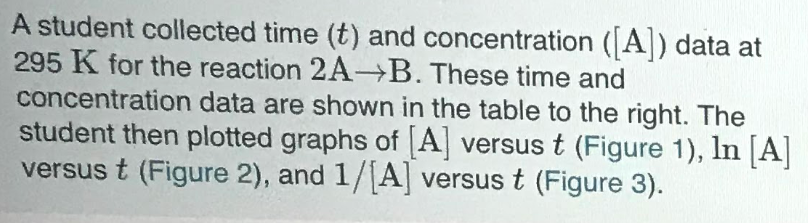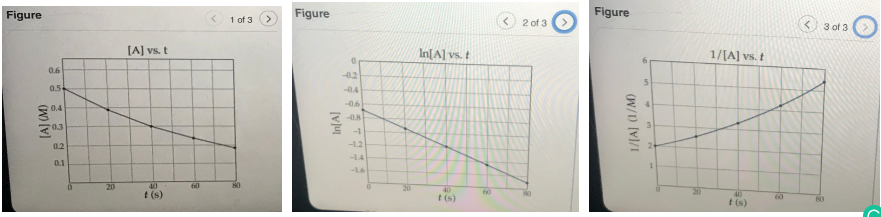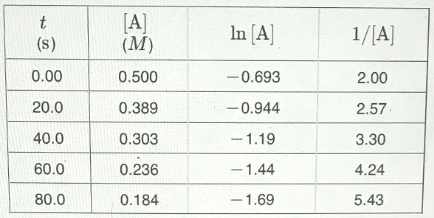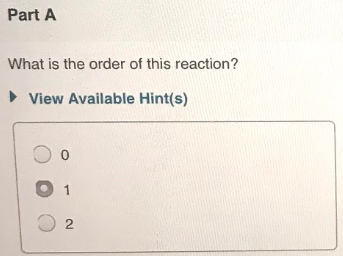# A student collected time(t) and concentration ([A]) data at 295 K for the reaction 2A →B. These time and concentration data are shown in the table to the right. The student then plotted graphs of {A] versus t (Figure 1), ln [A] versus t (Figure 2), and 1/[A] versus t (Figure 3). What is the order of this reaction? a) 0 b) 1 c) 2